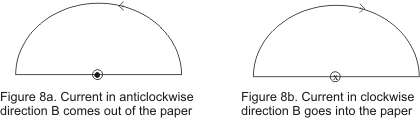# Applications of Biot Savart law

Table of Content

## Applications of Biot Savart law

Biot Savart law has many application In this section we will now apply Biot-Savart law as studied in previous section to calculate field B in some important cases

## (i) Magnetic Field due to steady current in an infinitely long straight wire

• Consider a straight infinitely long wire carrying a steady current I
• We want to calculate magnetic field at a point P at a distance R from the wire as shown below in figure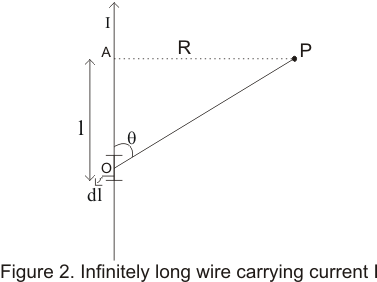• From Biot,-Savart law ,magnetic field dB due to small current element of the wire at point O at a distance |r|=r from point P is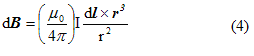• since current element Idl and vector r makes an angle θ with each other ,the magnitude of the product dlXr is dlrsinθ and is directed perpendicular to both dl and r vector as shown in the figure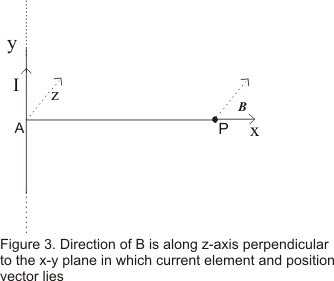• Since from our choice of co-ordinate, we found out that field B lies along z-axis therefore we can write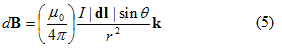where k is the unit vector along z-axis
• we will now express sinθ and r in terms of R which is fixed distance for any point in space and l which describes the position of current element on the infinitely long wire .From figure 1 we have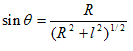and r=(R2 +l2)1/2
Putting these values in the equation (5) we find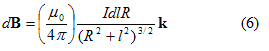• To find the field due to entire straight wire carrying wire ,we would have to integrate equation (6) B=∫dB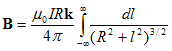• To evaluate the integral on the RHS substitute
l=RtanΦ and dl=Rsec2Φ dΦ
Therefore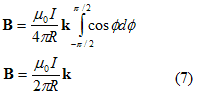• From equation (7) ,we noticed that
i) Magnetic field is proportional to the current I
ii) It is inversely proportional to the distance R
iii)Magnetic field is in the direction perpendicular to the straight wire and vector AP=R
• The magnetic line of force near a linear current carrying wire are concentric circles around the conductor in a plane perpendicular to the wire
• Hence the direction of field Bat point P at a distance R from wire, will be along the tangent drawn on a circle of radius R around the conductor as shown below in figure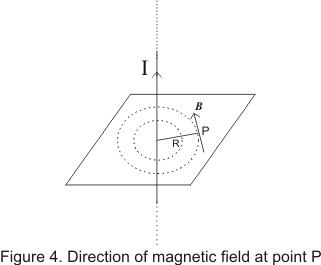Direction of B
• Direction of B can be found by right hand thumb rule i.e. grasp the wire with right hand ,the thumb pointing in direction of current ,the finger will curl around the wire in the direction of B
• The magnetic field lines are circular closed curve around the wire

## (ii) Force between two long and parallel current carrying conductor

• It is experimentally established fact that two current carrying conductors attract each other when the current is in same direction and repel each other when the current are in opposite direction
• Figure below shows two long parallel wires separated by distance d and carrying currents I1 and I2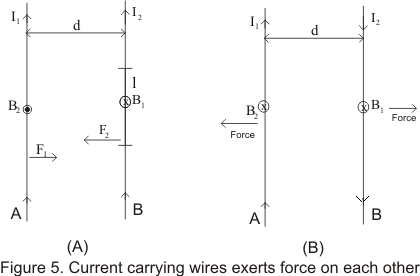• Consider fig 5(a) wire A will produce a field B1 at all near by points .The magnitude of B1 due to current I1 at a distance d i.e. on wire b is
B10I1/2πd                   ----(8)
• According to the right hand rule the direction of B1 is in downward as shown in figure (5a)
• Consider length l of wire B and the force experienced by it will be (I2lXB) whose magnitude is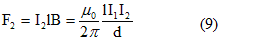• Direction of F2 can be determined using vector rule .F2 Lies in the plane of the wires and points to the left
• From figure (5) we see that direction of force is towards A if I2 is in same direction as I1 fig( 5a) and is away from A if I2 is flowing opposite to I1 (fig 5b)
• Force per unit length of wire B is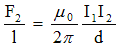• Similarly force per unit length of A due to current in B is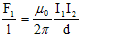and is directed opposite to the force on B due to A. Thus the force on either conductor is proportional to the product of the current
• We can now make a conclusion that the conductors attract each other if the currents are in the same direction and repel each other if currents are in opposite direction

## (iii) Magnetic Field along axis of a circular current carrying coil

• Let there be a circular coil of radius R and carrying current I. Let P be any point on the axis of a coil at a distance x from the center and which we have to find the field
• To calculate the field consider a current element Idl at the top of the coil pointing perpendicular towards the reader
• Current element Idl and r is the vector joining current element and point P as shown below in the figure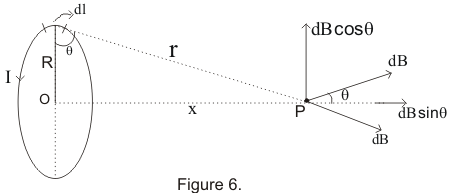• From Biot Savart law, the magnitude of the magnetic field due to this current element at P is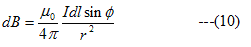where Φ is the angle between the length element dl and r
• Since Idl and r are perpendicular to each other so Φ=90.Therefore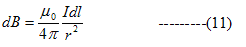• Resolving dB into two components we have dBsinθ along the axis of the loop and another one is dBcosθ at right angles to the x-axis
• Since coil is symmetrical about x-axis the contribution dB due to the element on opposite side ( along -y axis ) will be equal in magnitude but opposite in direction and cancel out. Thus we only have dBsinθ component
• The resultant B for the complete loop is given by,
B=∫dB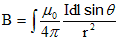Now from figure 6
sinθ=R/r =R/√(R2 + x2) So
eq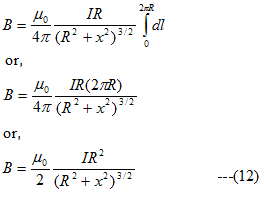• If the coil has N number of turns then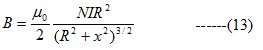Direction of B

• Direction of magnetic field at a point on the axis of circular coil is along the axis and its orientation can be obtained by using right hand thumb rule .If the fingers are curled along the current, the stretched thumb will point towards the magnetic field
• Magnetic field will be out of the page for anti-clockwise current and into the page for clockwise direction
Field at center of the coil

• At the center of the coil x=0
so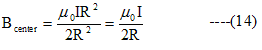Field at point far away from the center x>>>R

• In this case R in the denominator can be neglected hence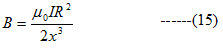• For coil having N number of turns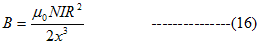• If the area of the coil is πR2 then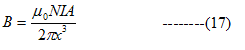• m=NIA represents the magnetic moment of the current coil. Thus from equation (17) we have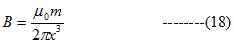## (iv) Magnetic Field at the center of a current carrying arc

• Consider an arc of radius R carrying current I as shown below in the figure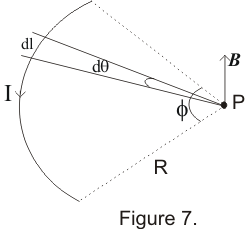• According to the Biot Savart law the magnetic field at any point P is given by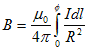Here dl=RdΦ
So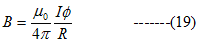• If l is the length of the arc then
l=RΦ so that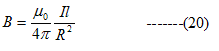• Equation 19 and 20 gives us magnetic field only at the center of curvature of a circular arc of current
• For semi circular loop put Φ=π in equation 19 and for full circle Φ=2π in equation 19 and calculate to find the result
• If the circular current loop lies on the plane of the paper then magnetic field will be out of the page for anticlockwise current and into the page for clockwise current as shown below in the figure